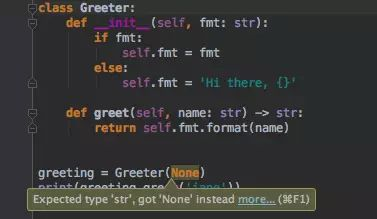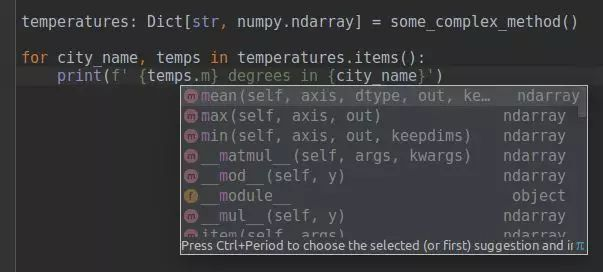# 在Python 2.7即将停止支持时，我们为你准备了一份3.x迁移指南

Python 3 功能简介

Python 是机器学习和其他科学领域中的主流语言，我们通常需要使用它处理大量的数据。Python 兼容多种深度学习框架，且具备很多优秀的工具来执行数据预处理和可视化。

pathlib 是 Python 3 的默认模块，帮助避免使用大量的 os.path.joins：

``````from pathlib import Path
dataset = 'wiki_images'
datasets_root = Path('/path/to/datasets/') train_path = datasets_root / dataset / 'train'
test_path = datasets_root / dataset / 'test'for image_path in train_path.iterdir():with image_path.open() as f: # note, open is a method of Path object# do something with an image
``````

Python 2 总是试图使用字符串级联（准确，但不好），现在有了 pathlib，代码安全、准确、可读性强。

``````p.exists()
p.is_dir()
p.parts()
p.with_name('sibling.png') # only change the name, but keep the folder
p.with_suffix('.jpg') # only change the extension, but keep the folder and the name
p.chmod(mode)
p.rmdir()
``````

pathlib 会节约大量时间，详见：

• 文档：docs.python.org/3/libra
• 参考信息：pymotw.com/3/pathlib/

PyCharm 中的类型提示示例：Python 不只是适合脚本的语言，现在的数据流程还包括大量步骤，每一步都包括不同的框架（有时也包括不同的逻辑）。

``````def repeat_each_entry(data):""" Each entry in the data is doubled <blah blah nobody reads the documentation till the end>"""index = numpy.repeat(numpy.arange(len(data)), 2)return data[index]
``````

``````repeat_each_entry(pandas.Series(data=[0, 1, 2], index=[3, 4, 5])) # returns Series with Nones inside
``````

``````def repeat_each_entry(data: Union[numpy.ndarray, bcolz.carray]):
``````

``````@enforce.runtime_validation
def foo(text: str) -> None:
print(text)foo('Hi') # ok
foo(5) # fails@enforce.runtime_validation
def any2(x: List[bool]) -> bool:
return any(x)any ([False, False, True, False]) # True
any2([False, False, True, False]) # Trueany (['False']) # True
any2(['False']) # failsany ([False, None, "", 0]) # False
any2([False, None, "", 0]) # fails
``````

``````# Python 3
from astropy import units as u
@u.quantity_input()
def frequency(speed: u.meter / u.s, wavelength: u.m) -> u.terahertz:
return speed / wavelengthfrequency(speed=300_000 * u.km / u.s, wavelength=555 * u.nm)
# output: 540.5405405405404 THz, frequency of green visible light
``````

``````# l2-regularized linear regression: || AX - b ||^2 + alpha * ||x||^2 -> min# Python 2
X = np.linalg.inv(np.dot(A.T, A) + alpha * np.eye(A.shape)).dot(A.T.dot(b))
# Python 3
X = np.linalg.inv(A.T @ A + alpha * np.eye(A.shape)) @ (A.T @ b)
``````

``````import glob# Python 2
found_images = \glob.glob('/path/*.jpg') \
+ glob.glob('/path/*/*.jpg') \
+ glob.glob('/path/*/*/*.jpg') \
+ glob.glob('/path/*/*/*/*.jpg') \
+ glob.glob('/path/*/*/*/*/*.jpg') # Python 3
found_images = glob.glob('/path/**/*.jpg', recursive=True)
``````

python3 中更好的选择是使用 pathlib：

``````# Python 3
found_images = pathlib.Path('/path/').glob('**/*.jpg')
``````

Print 在 Python3 中是函数

Python 3 中使用 Print 需要加上麻烦的圆括弧，但它还是有一些优点。

``````print >>sys.stderr, "critical error" # Python 2
print("critical error", file=sys.stderr) # Python 3
``````

``````# Python 3
print(*array, sep='\t')
print(batch, epoch, loss, accuracy, time, sep='\t')
``````

``````# Python 3
_print = print # store the original print function
def print(*args, **kargs):
pass # do something useful, e.g. store output to some file
``````

``````@contextlib.contextmanager
def replace_print():
import builtins_print = print # saving old print function
# or use some other function herebuiltins.print = lambda *args, **kwargs: _print('new printing', *args, **kwargs)
yieldbuiltins.print = _printwith replace_print():
<code here will invoke other print function>
``````

print 函数可以加入列表解析和其它语言构建结构。

``````# Python 3
result = process(x) if is_valid(x) else print('invalid item: ', x)
``````

f-strings 可作为简单和可靠的格式化

``````# Python 2
print('{batch:3} {epoch:3} / {total_epochs:3}  accuracy: {acc_mean:0.4f}±{acc_std:0.4f} time: {avg_time:3.2f}'.format(batch=batch, epoch=epoch, total_epochs=total_epochs, acc_mean=numpy.mean(accuracies), acc_std=numpy.std(accuracies),avg_time=time / len(data_batch)
))# Python 2 (too error-prone during fast modifications, please avoid):
print('{:3} {:3} / {:3}  accuracy: {:0.4f}±{:0.4f} time: {:3.2f}'.format(batch, epoch, total_epochs, numpy.mean(accuracies), numpy.std(accuracies),time / len(data_batch)
))
``````

``````120 12 / 300  accuracy: 0.8180±0.4649 time: 56.60
``````

f-strings 即格式化字符串在 Python 3.6 中被引入：

``````# Python 3.6+
print(f'{batch:3} {epoch:3} / {total_epochs:3}  accuracy: {numpy.mean(accuracies):0.4f}±{numpy.std(accuracies):0.4f} time: {time / len(data_batch):3.2f}')
``````

``````query = f"INSERT INTO STATION VALUES (13, '{city}', '{state}', {latitude}, {longitude})"
``````

「true division」和「integer division」之间的明显区别

``````data = pandas.read_csv('timing.csv')
velocity = data['distance'] / data['time']
``````

Python 2 中的结果依赖于『时间』和『距离』（例如，以米和秒为单位）是否被保存为整数。

``````n_gifts = money // gift_price  # correct for int and float arguments
``````

``````# All these comparisons are illegal in Python 3
3 < '3'
2 < None
(3, 4) < (3, None)
(4, 5) < [4, 5]# False in both Python 2 and Python 3
(4, 5) == [4, 5]
``````

``````sorted([2, '1', 3]) # invalid for Python 3, in Python 2 returns [2, 3, '1']
``````

``````if a is not None:
pass
if a: # WRONG check for None
pass
``````

``````s = '您好'
print(len(s))
print(s[:2])
``````

• Python 2: 6\n��
• Python 3: 2\n 您好.
``````x = u'со'
x += 'co' # ok
x += 'со' # fail
``````

Python 2 在此失败了，而 Python 3 可以如期工作（因为我在字符串中使用了俄文字母）。

``````'a' < type < u'a' # Python 2: True
'a' < u'a' # Python 2: False
``````

``````from collections import Counter
Counter('Möbelstück')
``````
• Python 2: `Counter({'\xc3': 2, 'b': 1, 'e': 1, 'c': 1, 'k': 1, 'M': 1, 'l': 1, 's': 1, 't': 1, '\xb6': 1, '\xbc': 1})`
• Python 3: `Counter({'M': 1, 'ö': 1, 'b': 1, 'e': 1, 'l': 1, 's': 1, 't': 1, 'ü': 1, 'c': 1, 'k': 1})`

``````import json
x = {str(i):i for i in range(5)}
# Python 2
{u'1': 1, u'0': 0, u'3': 3, u'2': 2, u'4': 4}
# Python 3
{'0': 0, '1': 1, '2': 2, '3': 3, '4': 4}
``````

``````from torch import nn# Python 2
model = nn.Sequential(OrderedDict([
('conv1', nn.Conv2d(1,20,5)),
('relu1', nn.ReLU()),
('conv2', nn.Conv2d(20,64,5)),
('relu2', nn.ReLU())
]))# Python 3.6+, how it *can* be done, not supported right now in pytorch
model = nn.Sequential(conv1=nn.Conv2d(1,20,5),relu1=nn.ReLU(),conv2=nn.Conv2d(20,64,5),relu2=nn.ReLU())
)
``````

``````# handy when amount of additional stored info may vary between experiments, but the same code can be used in all cases
model_paramteres, optimizer_parameters, *other_params = load(checkpoint_name)# picking two last values from a sequence
*prev, next_to_last, last = values_history# This also works with any iterables, so if you have a function that yields e.g. qualities,
# below is a simple way to take only last two values from a list
*prev, next_to_last, last = iter_train(args)
``````

``````# Python 2
import cPickle as pickle
import numpy
print len(pickle.dumps(numpy.random.normal(size=[1000, 1000])))
# result: 23691675# Python 3
import pickle
import numpy
len(pickle.dumps(numpy.random.normal(size=[1000, 1000])))
# result: 8000162
``````

``````labels = <initial_value>
predictions = [model.predict(data) for data, labels in dataset]# labels are overwritten in Python 2
# labels are not affected by comprehension in Python 3
``````

Python 2 的 super（...）是代码错误中的常见原因。

``````# Python 2
class MySubClass(MySuperClass):
def __init__(self, name, **options):super(MySubClass, self).__init__(name='subclass', **options)# Python 3
class MySubClass(MySuperClass):
def __init__(self, name, **options):super().__init__(name='subclass', **options)
``````

• 以清晰的形式写下你的期望
• 从 IDE 获取良好的建议``````x = dict(a=1, b=2)
y = dict(b=3, d=4)
# Python 3.5+
z = {**x, **y}
# z = {'a': 1, 'b': 3, 'd': 4}, note that value for `b` is taken from the latter dict.
``````

aame 方法对于列表（list）、元组（tuple）和集合（set）都是有效的（a、b、c 是任意的可迭代对象）：

``````[*a, *b, *c] # list, concatenating
(*a, *b, *c) # tuple, concatenating
{*a, *b, *c} # set, union
``````

``````Python 3.5+
do_something(**{**default_settings, **custom_settings})# Also possible, this code also checks there is no intersection between keys of dictionaries
do_something(**first_args, **second_args)
``````

``````model = sklearn.svm.SVC(2, 'poly', 2, 4, 0.5）
``````

``````class SVC(BaseSVC):
def __init__(self, *, C=1.0, kernel='rbf', degree=3, gamma='auto', coef0=0.0, ... )
``````
• 现在，用户需要明确规定参数 sklearn.svm.SVC(C=2, kernel='poly', degree=2, gamma=4, coef0=0.5) 的命名。
• 这种机制使得 API 同时具备了可靠性和灵活性。

``````# Python 3
math.inf # 'largest' number
math.nan # not a numbermax_quality = -math.inf  # no more magic initial values!for model in trained_models:max_quality = max(max_quality, compute_quality(model, data))
``````

Python 2 提供了两个基本的整数类型，即 int（64 位符号整数）和用于长时间计算的 long（在 C++变的相当莫名其妙）。

Python 3 有一个单精度类型的 int，它包含了长时间的运算。

``````isinstance(x, numbers.Integral) # Python 2, the canonical way
isinstance(x, (long, int)) # Python 2
isinstance(x, int) # Python 3, easier to remember
``````

• Enums 有理论价值，但是字符串输入已广泛应用在 python 数据栈中。Enums 似乎不与 numpy 交互，并且不一定来自 pandas。
• 协同程序也非常有希望用于数据流程，但还没有出现大规模应用。
• Python 3 有稳定的 ABI
• Python 3 支持 unicode（因此ω = Δφ / Δt 也 okay），但你最好使用好的旧的 ASCII 名称
• 一些库比如 jupyterhub(jupyter in cloud)、django 和新版 ipython 只支持 Python 3，因此对你来讲没用的功能对于你可能只想使用一次的库很有用。

``````map(lambda x, (y, z): x, z, dict.items())
``````

``````{x:z for x, (y, z) in d.items()}
``````

• map(), .keys(), .values(), .items(), 等等返回迭代器，而不是列表。迭代器的主要问题有：没有琐碎的分割和无法迭代两次。将结果转化为列表几乎可以解决所有问题。
• 遇到问题请参见 Python 问答：我如何移植到 Python 3？（eev.ee/blog/2016/07/31/

• 课程作者应该首先花时间解释什么是迭代器，为什么它不能像字符串那样被分片/级联/相乘/迭代两次（以及如何处理它）。
• 我相信大多数课程作者很高兴避开这些细节，但是现在几乎不可能。

Python 2 与 Python 3 共存了近 10 年，时至今日，我们必须要说：是时候转向 Python 3 了。